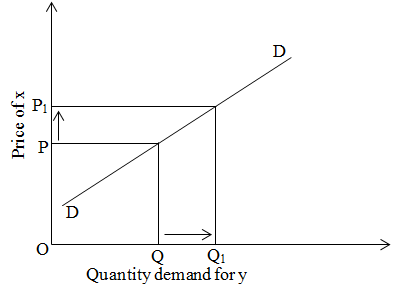# Positive cross elasticity of substitute goods

Cross elasticity of demand is positive because when the price of one commodity i.e. x commodity increases demand for another commodity i.e. y also increases. We can express by the help of given figure.On the above figure, in initial stage price of x commodity is OP and demand for y commodity is OQ. When price of x is increased from p to P1 then quantity demand for y commodity increases from Q to Q1.